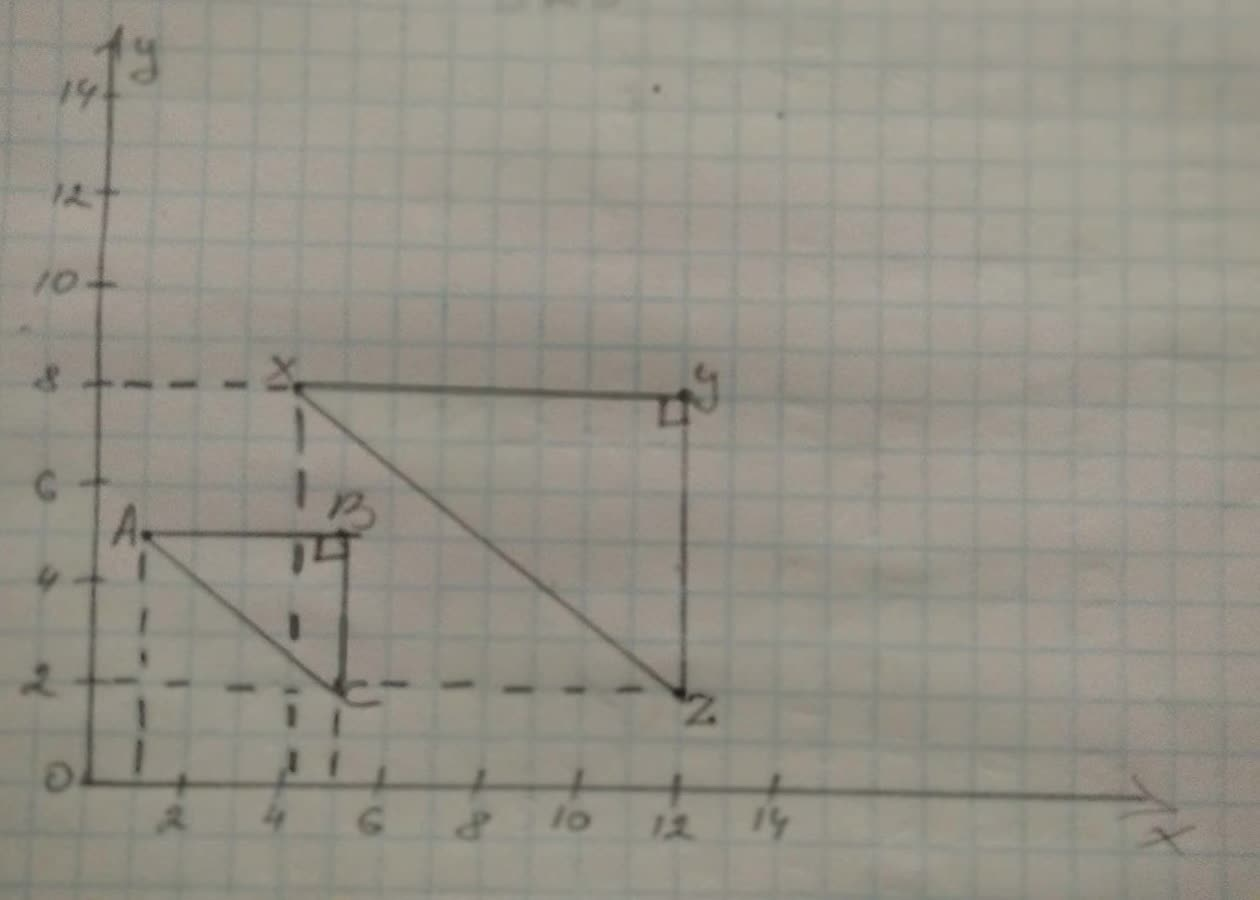## Triangles ABC and XYZ are shown in the coordinate plane below.

TrigonometryTriangles ABC and XYZ are shown in the coordinate plane below.2 years ago
Triangles ABC and XYZ are shown in the coordinate plane below. Are triangles ABC and XYZ similar? (Justify your answer using one or more transformations.)
Sol qn: △ ABC AB=4 BC=3 AC=sqrt(4^(2)+3^(2)) =5 Now qn: △ XYZ, (△ ABC and △ XYZ) XY=12-4=8=2AB are angle Y2=8-2=6=2BC △ x2=sqrt(8^(2)+6^(2))= 20=2AC △ ABC and △ XYZ (XY)/(AB)=2,(Y2)/(BC)=2,(XZ)/(AC)=2 arrow (XY)/(AB)=(YZ)/(BC)=(XZ)/(AC)=2 Since sides of corresponding triongles are proportional. △ ABC ~ △ XYZ (Bysss)